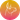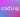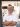• News
• Service
• Products
• Blog# BlogProgramming languages evolve towards always more abstract structures which help developers to reason about their code. Many structures are close to our natural way of thinking : objects, functions, data collections, etc. Monoids are a bit more abstract but once we recognize them, they become very useful.

### What is a monoid?

Mathematically, and keeping it short, a monoid is an algebraic structure that satisfies identity element and associativity.

So, let’s assume we have a type T and a binary operation op: `op(T,T) → T`, then T with op is a monoid if

• op is associative, for a, b, c in T, which means `op(op(a , b) , c) = op(a , op(b , c))`
• T has an identity element which we call neutral on op like: `op(neutral , a) = op(a, neutral) = a`

We call the identity element neutral, sometimes it's called zero and in some collection methods it's just z.

Sometimes operation is called combine or append, because it's usually the kind of thing operation will do.

In Scala we can define a monoid trait:

``````trait Monoid[A] {
def operation(a: A, b: A): A
def neutral : A
}``````

Let's take a few examples of monoids.

We can define the addition of integers as a monoid:

``````val intAddMonoid = new Monoid[Int] {
override def operation(i1: Int, i2: Int) = i1 + i2
override val neutral = 0
}``````

Or the concatenation of strings:

``````val stringConcatMonoid = new Monoid[String] {
override def operation(s1: String, s2: String) = s1 + s2
override val neutral = ""
}``````

Or the multiplication of integers:

``````  val intMultMonoid = new Monoid[Int] {
override def operation(i1: Int, i2: Int) = i1 * i2
override val neutral = 1
}``````

In the three cases, the two monoid laws hold and are easy to verify. Associativity is mathematically true for addition and multiplication, their identity elements, respectively 0 and 1, are obvious. For string, it seems even more obvious.

However, we could also define a monoid for subtraction, but that would be wrong:

``````  val intSubNotMonoid = new Monoid[Int] {
override def operation(i1: Int, i2: Int): Int = i1 - i2
override def neutral: Int = 0
}``````

intSubNotMonoid is not a monoid because the subtraction operation is mathematically not associative: 10 - (6 -3) ≠ (10 - 6) - 3

So calling it a monoid does not make it a monoid. We always have to make sure the implementing operation and the identity element hold the monoid laws. A good test suit should make sure it's all fine.

### But what can we do with monoids?

As for any abstract structure, recognizing it, is the first step. Once we start seeing monoids, we figure out they are almost everywhere. They become good friends to clean-up or factorize code. We see them a lot when we are working with collections and they are also very powerful abstractions when it comes to parallel programming.

#### In collections

The fold method from List which is implemented as a foldLeft in TraversableOnce expects a neutral z element and an associative binary operator... Sounds like a monoid isn't it?

And if we consider the signatures of foldLeft and foldRight and restrict their arguments to the same type, say A, we end-up with

``````foldRight[B](z: A)(op: (A, A) => A): A
foldLeft[B](z: A)(op: (A, A) => A): A``````

It seems like a good fit for monoids. So now we can do something like that:

``````val l =  1 :: 2 :: 4 :: 8 :: 16 :: Nil
println(l.fold(intMultMonoid.neutral)(intMultMonoid.operation))
println(l.foldRight(intMultMonoid.neutral)(intMultMonoid.operation))
println(l.foldLeft(intMultMonoid.neutral)(intMultMonoid.operation))``````

Clean code which is guaranteed to always produce the same results as long as the given monoid is indeed a monoid!

We can then create some helper object:

``````object MonoidHelpers {
def monoidFold[T](l: List[T], m: Monoid[T]): T = l.foldLeft(m.neutral)(m.operation)
}``````

and then simplify the previous calls even more:

``println(MonoidHelpers.monoidFold(l, intMultMonoid)``

#### For parallel programming

The fact that an operation is associative makes it a good candidate for parallel computation. Let's take our previous list and the multiplication monoid.

Folding means something like that:

``mult(mult(mult(mult(1 , 2) , 4) , 8) , 16)``

That's sequential. However, as mult is associative, we can also do any combination, for example:

``mult(mult(1 , 2) , mult(mult(4 , 8) , 16))``

And the result is always the same. So, we could run those calculations in parallel on as many separate threads as there would be available. For that, we can safely use the parallel version of the collection library:

`` def monoidFoldPar[T](l: List[T], m: Monoid[T]): T = l.par.foldLeft(m.neutral)(m.operation)``

The parallel collection library is very well explained in the scala docs.

Behind the scene, par splits the collection and then combines results automatically. Depending on the collection type, the splitters work differently. The results cannot rely on how the collections are splitted because it's not deterministic, therefore the need of associativity and the importance of monoids.

#### Building-up monoids

Monoids are also like lego briks, we can compose them easily. From two monoids we can get a tuple of monoids:

`````` def tupleMonoid[T1,T2](m1: Monoid[T1], m2: Monoid[T2]): Monoid[(T1,T2)] =
new Monoid[(T1,T2)] {
override val neutral = (m1.neutral, m2.neutral)

override def operation(a: (T1, T2), b: (T1, T2)): (T1, T2) = {
(m1.operation(a._1, b._1), m2.operation(a._2, b._2))
}
}``````

Now we can combine two monoid calculations at the same time. We would need to give it the two monoids and would get the tuple back.

##### Merging two maps

An interesting use case is merging two maps and applying a function to the values when the keys are identical. Let's take two maps, the values could be the occurences of the words in some corpus.

``````val m1 = Map("hello" -> 11, "earth" -> 3, "ra" -> 17, "here" -> 5, "home" -> 10, "house" -> 2)
val m2 = Map("here" -> 13, "hello" -> 14, "house" -> 4, "waouh" -> 15, "ra" -> 4, "yyy" -> 8)``````

A simple merge version applying a passed function to the values:

``````def mapMergeSimple[K, V](a: Map[K, V], b: Map[K, V])(f: (V, V) ⇒ V): Map[K, V] = {
a ++ b.map {
case (k, v) =>
k -> (if (a.isDefinedAt(k)) f(v, a(k)) else v)
}
}``````

Another way to do it is with a Monoid. The code looks a bit more complicated but later on it's going to be easier to use because we can further compose it.

``````def mapMergeM[K, V](m: Monoid[V]): Monoid[Map[K, V]] =
new Monoid[Map[K, V]] {
override def neutral: Map[K, V] = Map.empty
override def operation(l: Map[K, V], r: Map[K, V]): Map[K, V] =
(l.keySet ++ r.keySet).foldLeft(neutral) {
case (res, key) =>
res.updated(key,
m.operation(l.getOrElse(key, m.neutral), r.getOrElse(key, m.neutral))
)
}
}``````

And finally, let's run the code:

``````println(mapMergeM(MyGreatMonoids.intAddMonoid).operation(m1, m2))
println(mapMergeSimple(m1, m2)((i1, i2) ⇒ i1 + i2))``````

produces:

``````Map(home -> 10, here -> 18, ra -> 21, house -> 6, waouh -> 15, yyy -> 8, hello -> 25, earth -> 3)
Map(home -> 10, here -> 18, ra -> 21, house -> 6, waouh -> 15, yyy -> 8, hello -> 25, earth -> 3)``````

It's worth mentioning that the scalaz library is implementing many algebraic structures keeping very close to their mathematical counterparts. Thus, in scalaz, Monoid extends Semigroup. Semigroup provides the binary associative operation and Monoid adds the identity element.

Thanks for reading and enjoy your daily Scala programming.26 Apr 2019